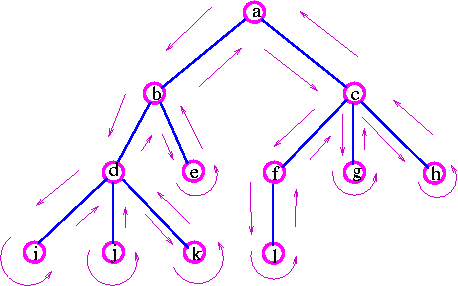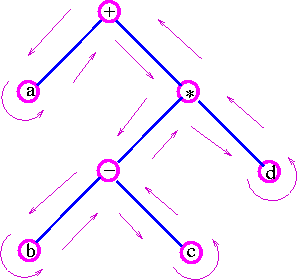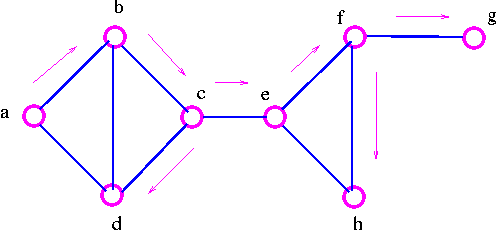Graph Theory

Tree Searching

There are two well-known search methods. They are brown as depth-first search( DFS) and breadth-first search (BFS). Each of these methods lists the vertices as they are encountered, and indicates the direction in which each edge is first traversed. The methods differ only in the way in which the vertex-lists are constructed.

Depth-First Search (DFS)

The basic idea of depth-first search is to penetrate as deeply as possible into a tree before fanning out to other vertices. This idea may be depicted as follows:a -> b --> d --> i -> j -> k -> e -> c -> f -> i -> g  -> h.

Another example, the expression a + {(b-c) × d} can represented by following tree:Example: Consider the graphNote that we have marked those edges we used when going to new vertex. These edges form a spanning tree, called a DFS spanning tree.

The basic idea of breadth-first search is to fan out to as many vertices as possible before penetrating deep into a tree. This idea may be depicted as follows:a --> b --> c --> d --> e --> f --> g --> h --> i --> j --> k --> e

above example clearly shows that breadth-first search must complete each level before proceeding to the next one.

Example: Consider the graphNote that we have marked those edges we used when going to new vertex. These edges form a spanning tree, called a BFS spanning tree.